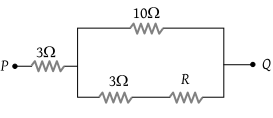NEET Physics Current Electricity Questions Solved

In the circuit shown here, what is the value of the unknown resistor R so that the total resistance of the circuit between points P and Q is also equal to(1) 3 ohms

(2) $\sqrt{39}\text{\hspace{0.17em}}ohms$

(3) $\sqrt{69}\text{\hspace{0.17em}}ohms$

(4) 10 ohms

Explanation is a part of a Paid Course. To view Explanation Please buy the course.

Difficulty Level: